# Test: Transpose Of A Matrix

## 10 Questions MCQ Test Mathematics (Maths) Class 12 | Test: Transpose Of A Matrix

Description
This mock test of Test: Transpose Of A Matrix for JEE helps you for every JEE entrance exam. This contains 10 Multiple Choice Questions for JEE Test: Transpose Of A Matrix (mcq) to study with solutions a complete question bank. The solved questions answers in this Test: Transpose Of A Matrix quiz give you a good mix of easy questions and tough questions. JEE students definitely take this Test: Transpose Of A Matrix exercise for a better result in the exam. You can find other Test: Transpose Of A Matrix extra questions, long questions & short questions for JEE on EduRev as well by searching above.
QUESTION: 1

### If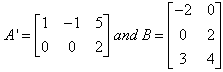, then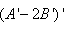is equal to

Solution:

A’ = {(1,-1,5) (0,0,2)}   B = {(-2,0) (0,2) (3,4)}
B’ = {(-2,0,3) (0,2,4)}
2B’ = 2{(-2,0,3) (0,2,4)}
2B’ = {(-4,0,6) (0,4,8)}
(A’ - 2B’) = {(1,-1,5) (0,0,2)}  - {(-4,0,6) (0,4,8)}
= {(5,-1,-1) (0,-4,-6)}
(A’ - 2B’)’ = {(5,0) (-1,-4) (-1,-6)}

QUESTION: 2

### If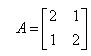, then (A2)`  is equal to

Solution:

A = {(2,1) (1,2)}
A2 =  {(2,1) (1,2)} * {(2,1) (1,2)}
A2 = {(4 + 1) (2 + 2)} {(2 + 2) (1 + 4)}
A2 = {5,4} {4,5}

QUESTION: 3

### The transpose of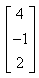is

Solution:
QUESTION: 4

If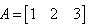and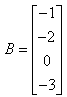then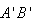is equal to

Solution:

A = {1, 2, 3}   B = {(-1), (-2), (0), (-3)}
A’ = {(1), (2), (3)}  B’ = {-1, -2, 0 , -3}
A’B’ = {(-1, -2, 0, 3) (-2, -4 , 0, -6) (-3, -6, 0, -9)}

QUESTION: 5

The number of all possible matrices of order 3×3 with each entry 0 or 1 is

Solution:

23x3=29=512.

The number of elements in a 3 X 3 matrix is the product 3 X 3=9.

Each element can either be a 0 or a 1.

Given this, the total possible matrices that can be selected is 29=512

QUESTION: 6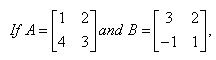, then (AxB) is equal to

Solution:

A ={(1,2) (4,3)} B = {(3,2) (-1,1)}
AB= {[(1*3)+(2*(-1)) (1*2)+(2*1)] [(4*3)+(3 *(-1)) (4*2)+(3*1)]}
= {(1,4) (9,11)}

QUESTION: 7

If A is a symmetric matrix, then B’ AB is​

Solution:

If A is a symmetric matrix, then B'AB is a symmetric metrix. So, B'AB is a symmetric matrix.

QUESTION: 8

The transpose of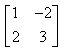is

Solution:

The transpose of a matrix is a new matrix whose rows are the columns of the original. (This makes the columns of the new matrix the rows of the original). Here is a matrix and its transpose: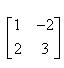is matrix and its transpose is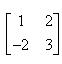QUESTION: 9

What is the maximum number of different elements needed to write a skew symmetric matrix of order n?​

Solution:

For a skew symmetric matrix , as we know all the diagonal elements are zero and the upper triangular elements are the same as that of lower triangular elements in such a fashion that the matrix A = -(transpose A) satisfies.

therefore , for a matrix A of dimension n *n , the diagonal elements are zero i.e there would be n zeros in the diagonal
no. of elements remaining to be distinct = total no. of elements -
diagonal elements = (n * n ) - n
= n2 - n

Now as we already said that the the upper traingular half elements are same as that of lower triangular half.

therefore the maximum number of distinct elements are                             = (n- n) /2

QUESTION: 10

If A, B are symmetric matrices of same order then the matrix AB-BA is a​

Solution:

A and B are symmetric matrices, therefore, we have:
A′=A and B′=B..........(i)

Consider
(AB−BA)′=(AB)′ − (BA)′,[∵(A−B)′=A′B′]

=B′A′− A′B',[∵(AB)′= B′A]

=BA−AB [by (i) ]

=−(AB−BA)

∴(AB−BA) ′=−(AB−BA)

Thus, (AB−BA) is a skew-symmetric matrix.